One of the most interesting series of questions on this year's Lesswrong survey were the calibration questions. This has been a staple of previous Lesswrong surveys. "Bayesianism" is an old trope of Lesswrong, and it's interesting to see how good we really are at estimating probabilities. Yvain was disappointed that Lesswrongers did not seem to be less overconfident, on previous surveys.

One of the problems with previous surveys is they only had a few questions. The 2012 survey used only a single question to estimate calibration, when Thomas Bayes was Born. A single question is totally useless. It assumes that people's predictions are totally independent of each other, and obviously they aren't. There could be a bias, where most people are overconfident on that particular question. But they could be underconfident on other questions. As long as the biases cancel out, they are perfectly calibrated. Adjusting their probability estimates down everywhere would not help them make better predictions.

This years survey had 8 questions:

1. Are you smiling right now?
2. Which is heavier, a virus or a prion?
3. I'm thinking of a number between one and ten, what is it?
4. What year was the fast food chain "Dairy Queen" founded? (Within five years)
5. Alexander Hamilton appears on how many distinct denominations of US Currency?
6. Without counting, how many keys on a standard IBM keyboard released after 1986, within ten?
7. What's the diameter of a standard soccer ball, in cm within 2?
8. How many calories in a Reese's peanut butter cup within 20?

What's interesting about these questions is a few of them are difficult to Google. At least they aren't the first search result, or the first search result isn't correct. There is also some controversy over the correct answers. Here are the answers:

1. True. No, really, I just marked any answer you put in there as correct, as long as it wasn't empty.
2. Virus. Although there was some dispute over that. 74% of people got this right. The median probability people gave to getting the answer right was 65%.
3. 4 - Ingres used a random number generator. 6% of people got this right. On median people expected to get this right with 10% probability (the average was 14%.)
4. 1940 - The median answer was 1955. 9% of people Got this right. Median probability was 10%.
5. 6 - Yes, seriously. No one got this question right. People expected to get this right with 50% probability.
6. 101 - This one is ambiguous. there are multiple IBM keyboards released in different countries and times. The US 1986 one has 101 keys, so I went with that. The median answer was 70. 27% of people got this right. The median probability estimate was 60%.
7. 22 - The median answer was 28. 20% of people got this right. Median probability estimate was 30%.
8. 105 - If you Google for this question, Google comes up with 87. But Reese's website claims 105. The median answer people gave was 120. 30% of people got this right. The median probability was 25%.

## Exploring the Data

Today I will give you more details than you ever wanted to know about how good Lesswrongers' are at estimating probability. This post is extremely experimental. The writing style, the content, and the format. I've never done anything like this before. I am trying the R programming language, as well as R markdown. R markdown is a tool for writing documents that include code and plots in the output, which is really cool. I hope to encourage people to copy my code and play with the survey data. Just install R Studio and you can jump right in.

### Getting the Data

So now let's just explore the data. First we need to download it. Also clean it up a bit. Removing missing values, convert columns to the right datatype, and remove some troll submissions. Boring stuff, you can skip this.

``````#Downloads the lesswrong survey data
if (!file.exists('2016_lw_survey_public_release_3.csv'))

#remove useless first 3 columns
survey <- survey[,-c(1:3)]

#replace missing data with NA
survey[ survey == '' ] <- NA
survey[ survey == 'N/A' ] <- NA

#identify the columns that are writeins, and converts them to character type instead of factor.
writeInColumns <- grep('writein|\\.other\\.', colnames(survey), ignore.case = TRUE)
survey[writeInColumns] <- lapply(survey[writeInColumns], as.character)

#convert select columns to numeric
numericCols <- c("IQ", "Age", "SAT", "SAT2", "ACT")
survey[numericCols] <- lapply(survey[numericCols], function(x){suppressWarnings(as.numeric(x))})

#obvious trolls are obvious
rowsToRemove <- c(1983, 471, 2378, 2233, 33)
survey <- survey[-rowsToRemove, ]

#remove 185 people who didn't even fill out the first few questions
survey <- survey[!(is.na(survey\$Age) & is.na(survey\$BirthSex) & is.na(survey\$Gender)), ]
#View(survey)``````

Now to get just the calibration questions and clean them up.

``````#gets the numbers of the columns related to calibration
calCols <- grep('CalibrationQuestions', colnames(survey), ignore.case = TRUE)

#convert to character and remove %'s
survey[calCols] <- lapply(survey[calCols], function(x){gsub('%', '', as.character(x))})

#get the set of calibration questions as it's own dataframe
calset <- survey[,calCols]

#convert select columns to numeric, and all the even columns
calset[c((1:8)*2, 7, 11, 13, 15)] <- lapply(calset[c((1:8)*2, 7, 11, 13, 15)], function(x){suppressWarnings(as.numeric(x))})

#convert probabilities in percents, to proper probabilities
calset[,1:8*2] <- calset[,1:8*2]/100

survey[,calCols] <- calset

#find rows that don't have missing data
calNotMissing <- Reduce('&', lapply(calset, function(x){!is.na(x) & x != ''}))

#make a list of whether or not they got the answers right (then converts it to matrix)
#Are you smiling right now?
rep_len(TRUE, nrow(calset)), #of course you are.
#Which is heavier, a virus or a prion?
(grepl('virus', calset[], ignore.case = TRUE) & !grepl('prion', calset[], ignore.case = TRUE)), #accouting for the various letter cases and other text that people put into the answer, but ignoring people who put both answers in.
#I'm thinking of a number between one and ten, what is it?
calset[] == '4' | grepl('^four', calset[], ignore.case = TRUE), #accounting for people who spelled "four" >(
#What year was the fast food chain "Dairy Queen" founded? (Within five years)
abs(calset[]-1940) <= 5, #tests that the correct answer is within 5 of the true answer.
#Alexander Hamilton appears on how many distinct denominations of US Currency?
calset[] == '6' | grepl('^six', calset[], ignore.case = TRUE),
#Without counting, how many keys on a standard IBM keyboard released after 1986, within ten?
abs(calset[]-101) <= 10, #this one is incredibly ambiguous. there are multiple IBM keyboards released in different countries and times. The US 1986 one has 101 keys, going with that.
#What's the diameter of a standard soccerball, in cm within 2?
abs(calset[]-22) <= 2,
#How many calories in a reese's peanut butter cup within 20?
abs(calset[]-105) <= 20 #Google says 87, Reeses says 105, going with 105, but who knows?
), unlist)
#Keep row and column names consistent

#set missing answers to have missing value

#the probabilities given are the even numbered columns
probs <- data.matrix(calset[,1:8*2])
colnames(probs) <- paste('ProbabilityCalibration', 1:8, sep='')

#Get only the answers where no data is missing
completeProbs <- probs[calNotMissing,]``````

### Exploring the Questions

Now let's start making plots. First, lets see the distribution of the number of questions people got correct:

``````#counts the number of correct questions, and tables it
questionsCorrect <- table(apply(completeAns, 1, sum))

barplot(questionsCorrect/nrow(completeAns)*100, xlab = "Number of Questions Correct Out of 8", ylab = "Percent of Survey Respondents")``````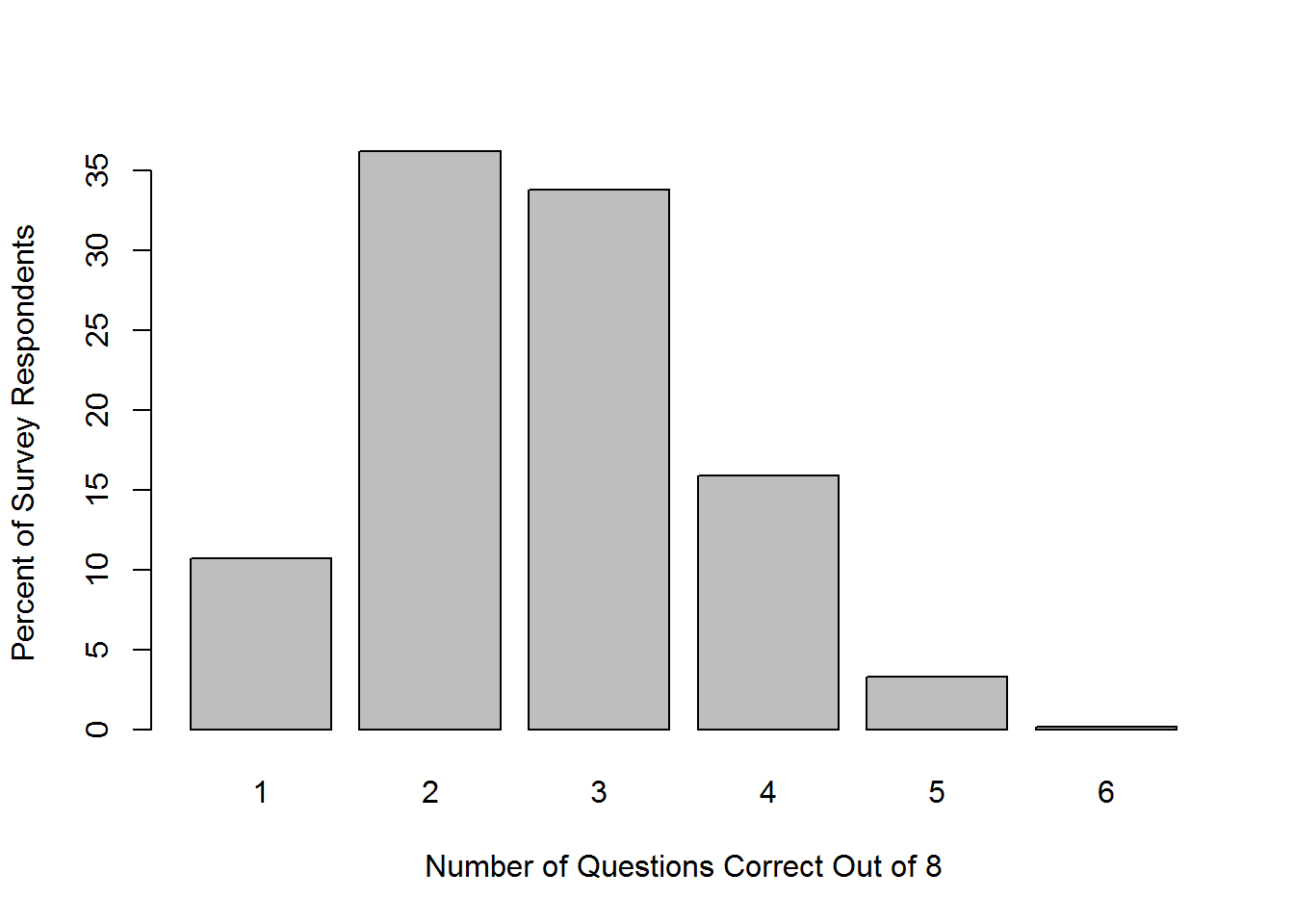Interestingly no one got all of the questions right. In fact no one even get 7 out of the 8 questions right, and only 2 got 6 out of 8. I'd expect there to be at least a few cheaters. It seems like Ingres asked questions that weren't easy to Google.

Also 10% of people got every question but the first wrong. Ouch. Most people only got 2 correct.

Lets see what specific questions people got wrong:

``````#the portion of people that got each question right.
probsEachQuestion <- apply(completeAns, 2, mean)

questions <- c('smiling', 'virus', 'guess number', 'dairy queen', 'Alexander Hamilton', 'keys on IBM keyboard', 'diameter of soccer ball', 'calories in reeses')
questions <- gsub(' ', '\n', questions)
par(mar = c(9,4,4,2) + 0.1)
barplot(probsEachQuestion, names.arg = questions, xlab = 'Question', ylab = 'Portion Correct', cex.names = 0.8, las=2, main="Difficulty Per Question")``````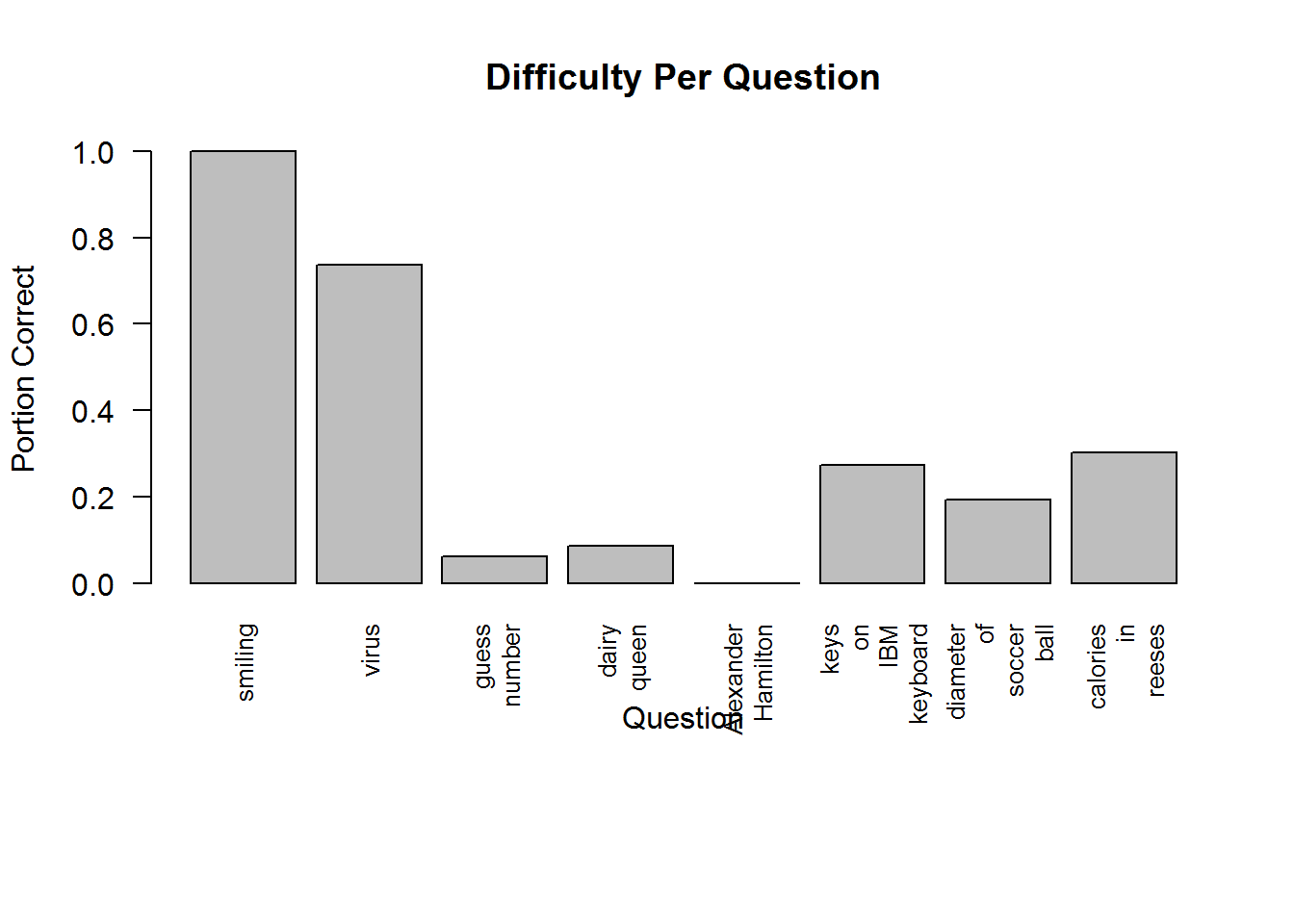Not a single person got the question about the number of bills Alexander Hamilton is on. But I think the other questions were about expected. Of course everyone got the smiling one correct by definition, it was just a baseline question.

Lets see what probability people expected to get each question correct:

``barplot(apply(completeProbs, 2, median), names.arg = questions, xlab = 'Question', ylab = 'Median Probability Estimate', cex.names = 0.8, las=2, main='Median Probability Estimate Per Question')``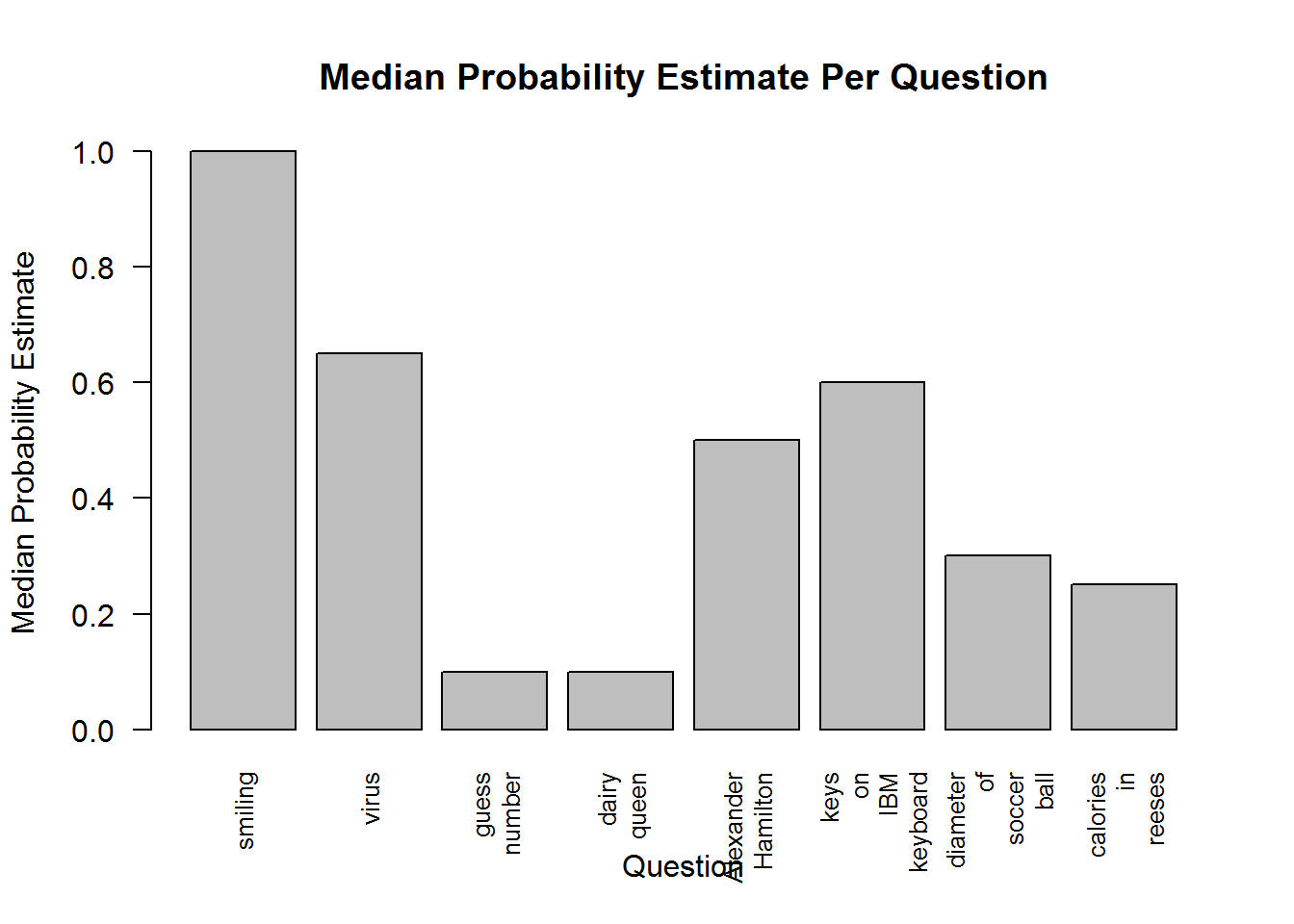Now look at the questions people were the most overconfident on. Here I plot the median probability estimate for each question, relative to the actual percent of people who got it correct.

``barplot(apply(completeProbs, 2, median)-probsEachQuestion, names.arg = questions, xlab = 'Question', ylab = 'Portion Correct', cex.names = 0.8, las=2, main='Difference Between Predicted Probability\nand Actual Probability')``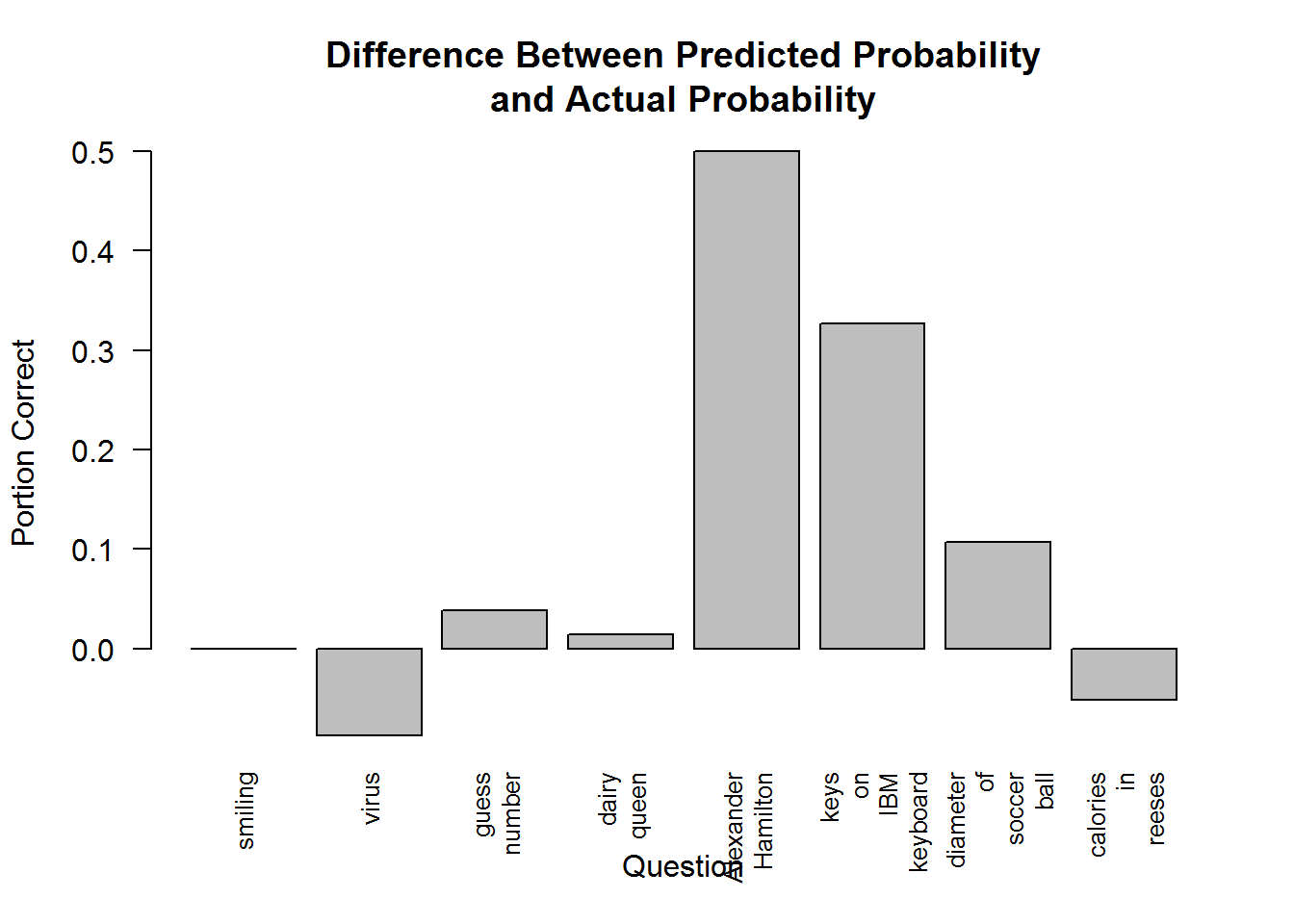The bars that go under the graph represent underconfidence. Everything else represents overconfidence. It seems like people were only (slightly) underconfident on two questions, and not particularly easy ones either.

### Exploring Calibration

OK now let's test calibration. What was the average portion of questions people got right?

``print(mean(apply(completeAns, 1, mean)))``
``##  0.3316379``

Now, what's the average probability people gave to the their answer being correct?

``print(median(apply(completeProbs, 1, mean)))``
``##  0.4437438``

So it looks like people are overconfident by about 11%. Wrong 1/10 times more than they expect to be wrong.

Let's sort people by the number of questions they got right, and the average probability they gave. E.g., of people who got 25% of questions right, how many questions did they expect to get right?

``````percentCorrect <- apply(completeAns, 1, mean)*100
averagePercent <- apply(completeProbs, 1, mean)*100
#I prefer median, but the result is almost identical with mean
probByCorrect <- tapply(averagePercent, percentCorrect, median)
names(probByCorrect) <- paste(names(probByCorrect), "%", sep='')
barplot(probByCorrect, xlab = 'Percent Correct', ylab = 'Median Probability Assigned (%)')``````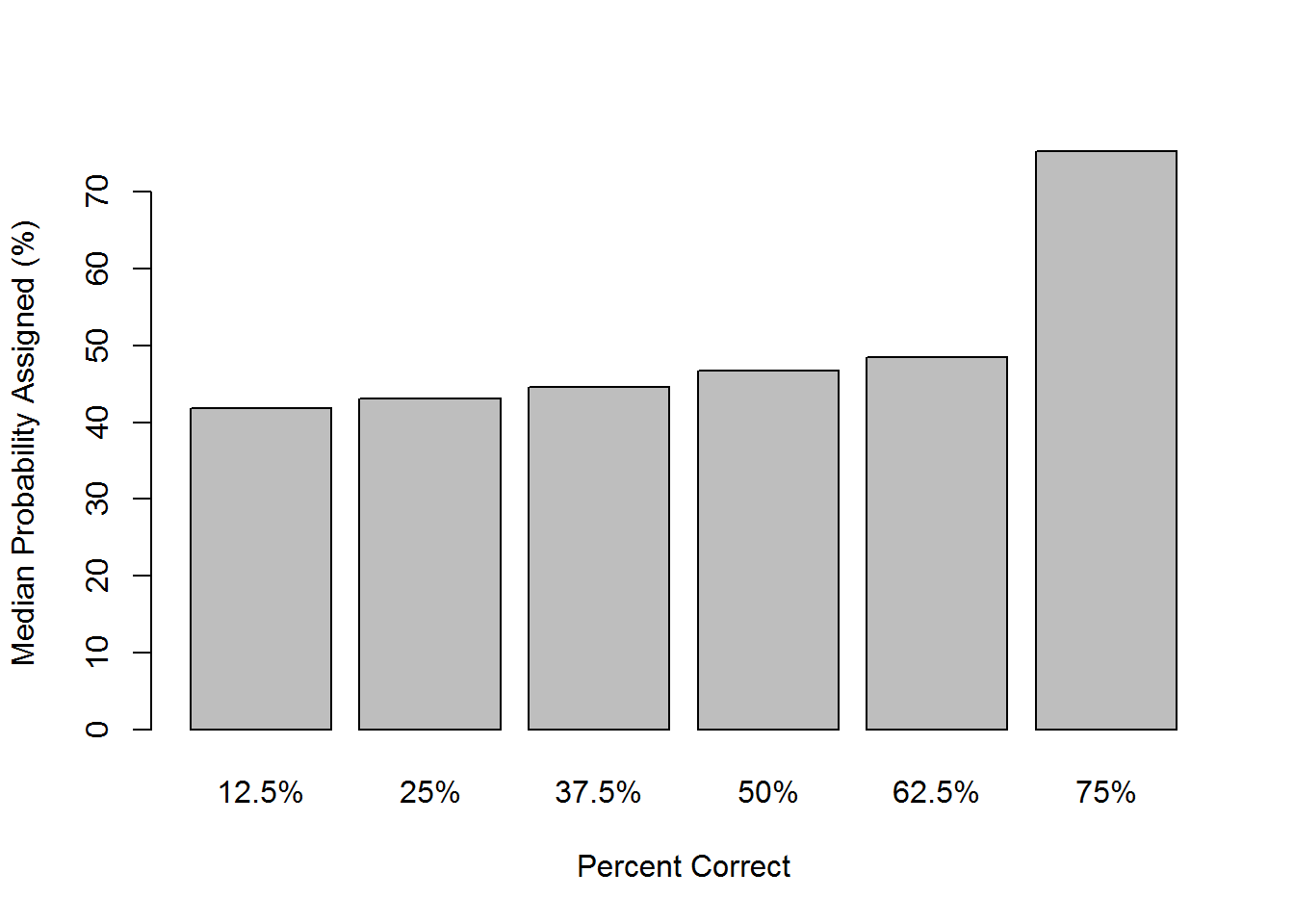Note that you can ignore the 75% category, there are only 2 people in it. Interesting that they are perfectly calibrated, but they are almost certainly cheating.

I'm really not sure what to make of this. It looks like people assigned the same probabilities regardless how many questions they got right. People who got only 1 question right assigned basically the same probability as those who got 5 right.

This could mean that everyone is very uncalibrated. But it could also suggest that basically everyone had the same probability of getting each question right, and so assigned basically the same probability. Some were just lucky, or unlucky. But they didn't expect to be lucky, so it isn't reflected in the median probability assignments.

Lets generate some artificial data from this model. That is assume everyone has exactly the same probability of getting each question correct. We will see if it matches our graph above, of the distribution of number of correct answers:

``````#Generates the random data
randomTest <- table(sapply(1:100000, function(x){min(sum(runif(8) < probsEachQuestion), 6)}))/100000*100
barplot(randomTest, xlab = "Number of Questions Correct Out of 8", ylab = "Percent of Random Tests", main="Randomly Generated Samples")``````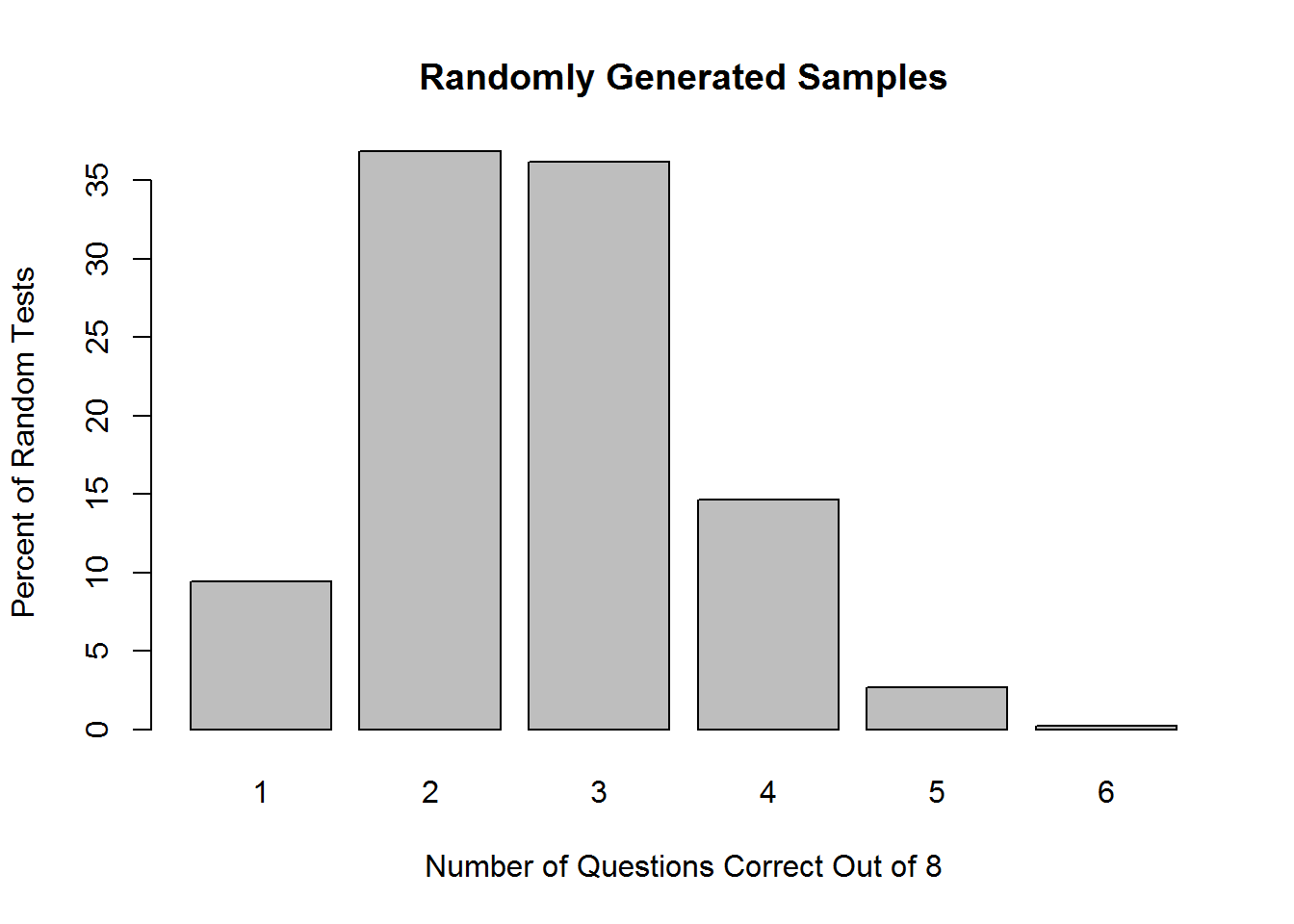That looks nearly identical to our plot above: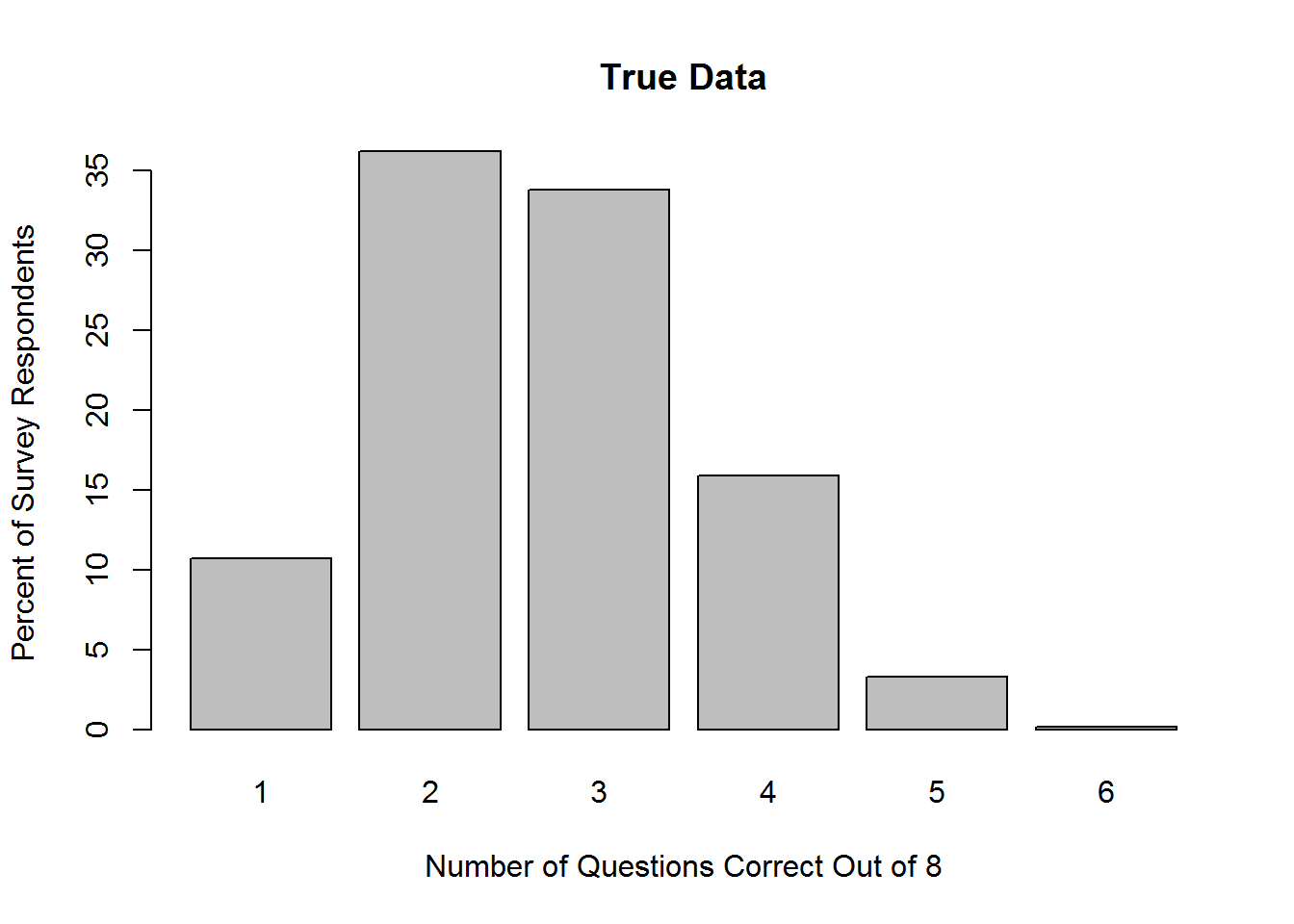Now let's look at the distribution of probabilities and see if that seems sensible:

``````#cuts the probs into intervals of 10% and counts the percent of people assigned values within them
probFactor <- cut(averagePercent, 10, include.lowest = TRUE)

levels(probFactor) <- paste(0:9*10, '%-', 1:10*10, '%', sep='')
tabledMeanProb <- table(probFactor)/length(probFactor)*100
barplot(tabledMeanProb, xlab = 'Average Percent Assigned\nto Calibration Quetions', ylab = 'Percent of Total Respondents', cex.names = 0.8, las=2)``````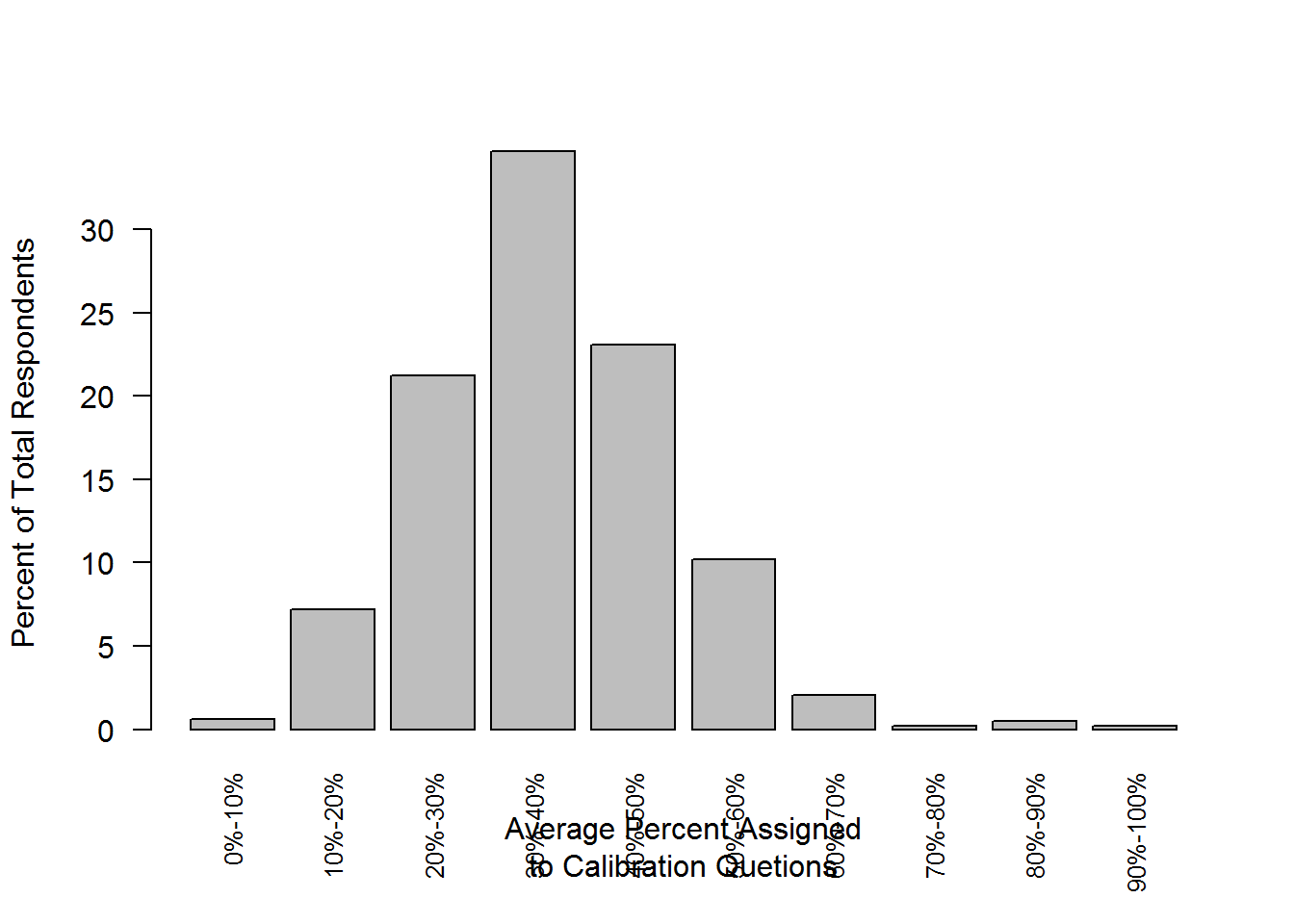So it looks like what we expected, most people gave an average probability assignment of 45%. A lot of people did give average probabilities much higher than that though. Let's see if those people were more likely to get more questions correct. According to our model, they should not be.

``barplot(tapply(percentCorrect, probFactor, mean), xlab = 'Average Percent Assigned\nto Calibration Quetions', ylab = 'Percent of Questions Correct', cex.names = 0.8, las=2)``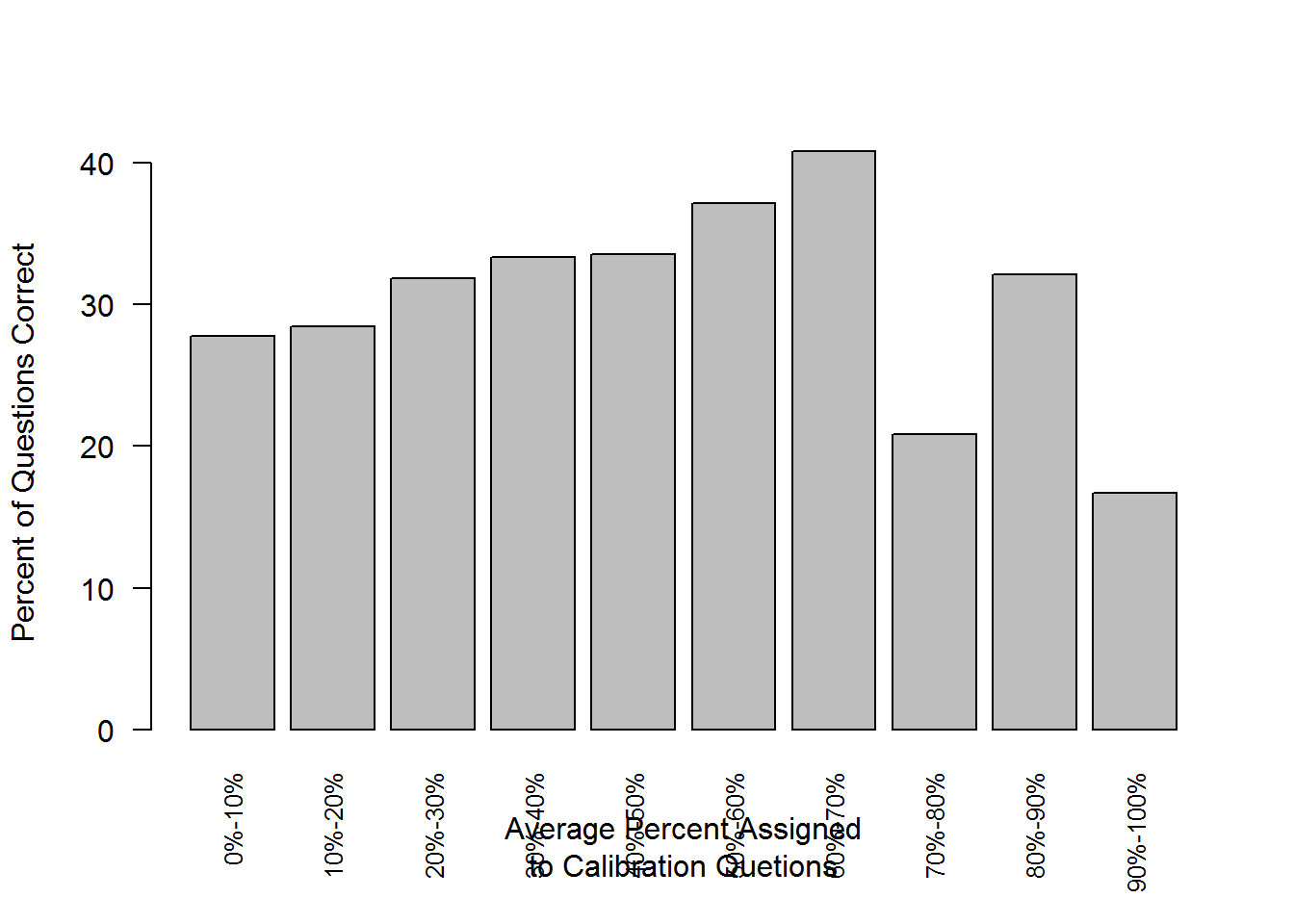Wow that doesn't look good! People who assigned significantly higher probabilities, were only slightly more likely to get them correct. In fact the extremely confident people were much less likely to be correct. But do note that there are only a handful of people (21) that were more than 70% confident.

But also, most who assigned low probabilities got more than expected right. In fact it looks like just about everyone got about the same percent right regardless what probability they assigned. So this fits the theory that most people had about the same chance (33%) of getting a random question correct. All that varies is calibration.

## Scoring Calibration

Coming up with a way to measure how "calibrated" an individual is, is surprisingly hard. In my brief research, I didn't find any established and obviously correct metrics.

The problem with existing calibration scores is some people might simply know more than others. Someone familiar with the metric system might be better at estimating the diameter of a soccer ball. Someone outside of the US probably has no idea when Dairy Queen was founded. We don't want to measure skill at trivia, we want to measure overconfidence.

### Geometric Mean of Likelihood (GML)

The first thing to try is "likelihood". This measures how much probability you assigned the actual outcome. So if you were predicting coin flips, each flip has 0.5 probability of being heads, and 0.5 probability of being tails. For 3 heads in a row, the probability is 0.5 * 0.5 * 0.5 or 0.5^3 . That's 0.125, or 13% probability that that specific outcome would occur. Which doesn't seem like much, but it's better than someone who assigned 0.3 probability to heads, but less than someone who assigned 0.75 probability.

One problem with this is that the number gets really small after even a few trials. It's also not very interpret able directly. We can solve this by taking the geometric mean. Just like the normal arithmetic mean is related to the sum of a set of numbers, the geometric mean is related to the product.

We use it because unlike the arithmetic mean, it is isomorphic to likelihood. Higher geometric mean means higher likelihood. Whereas with arithmetic mean, you can assign 0 probability to the actual outcome, and still get a high average. Geometric Mean of Likelihood heavily weights against that and would give you a score of 0.

``````#This works by adding numbers in the log domain, which is the same as multiplying them.
geoMean <- function(x) exp(mean(log(x)))

#this function returns the probabilities you assigned the actual outcome.
#E.g. if you predicted TRUE with 0.75 probability, and the outcome was FALSE, then it returns 0.25.
getProbAssigned <- function(Events, Probs) ifelse(Events, Probs, 1-Probs)

#only way to keep the metadata of Probs
Probs[ rep_len(TRUE, length(Probs)) ] <- mapply(getProbAssigned, Answers, Probs)
Probs
}

#print the median geometric mean of likilihood
print(median(getGML(completeAns, completeProbs)))``````
``##  0.6073704``

So the median Geometric Mean of Likelihood (GML from now on) is 0.61. That's like if you had a 61% chance of getting each question right, and assigned 61% probability to each. But it's still somewhat difficult to interpret this number. Let's compare it to a method that is perfectly calibrated, and has the same probability of getting each question right as the average survey taker:

``````#Imagine that you predict 80% probability to something that has 70% probability. So imagine 100 trials.
#In 70 trials the event would happen, and you would correctly predict the outcome with 80% probability.
#So that likelihood is 0.80x0.80x0.80... or  0.80^(0.7x100).
#In 30 trials, the event would fail to happen, and you would predict that outcome with only 20% probability.
#So the likelihood of that is 0.2^(0.3x100)
#The total likelihood of all 100 trials is 0.80^(0.7x100)x0.2^(0.3x100).
#Since I'm only doing 1 trial and not 100, I can replace the 100 with 1, and I get 0.8^(0.7)x(0.2)^(0.3)

likelihood <- function(estProb, trueProb) estProb^trueProb*(1-estProb)^(1-trueProb)
bestLikelihood <- geoMean(likelihood(probsEachQuestion, probsEachQuestion))
print(bestLikelihood)``````
``##  0.7054584``

This means that a perfectly calibrated person would have a GLM of 0.71. That doesn't mean they'd get the correct answer 71% of the time. Actually they'd only get it 33% of the time. But they would correctly predict whether or not their answer was correct 71% of the time.

It's important to remember that is what we are measuring here, not whether or not their answer was correct. Someone who got all the questions wrong, but also gave 0% probability, would be perfectly calibrated. And have a GML of 1! And that's fine. Calibration should measure how good you are at estimating probabilities, not at answering trivia.

However this method does reward that a bit. If you know for a fact when Dairy Queen was established, or how heavy prions are, you can get a better score than someone who is more calibrated, but doesn't know. It's not capturing just overconfidence like we want.

One way to get around this would be to figure out how far away we are from making optimal predictions. We could compute how much we would could adjust our predictions to have the highest likelihood. E.g. -10 could mean decrease every prediction by 10%, in order to get the highest likelihood.

The only problem with this, is it allows predictions to become greater than 100%, or less than 0%. This is really bad and causes errors. So first we need to map probabilities into a domain where it's impossible to have an invalid number.

Log odds have this property. Log odds are isomorphic to probabilities. Every real number corresponds to a valid probability. 0 corresponds to 50%, Infinity corresponds to 1, and -Infinity correspond to 0, and everything in between.

They also have a really nice property, in that adding log odds is equivalent to a Bayesian update. See here for more.

``````#first convert to odds.
#I.e. the portion of times an event will happen, for every time it doesn't happen.
odds <- function(p) p/(1-p)

#base 10 because it's more interpretable to humans, and has all the same properties.
logOdds <- function(p) log10(odds(p))

#undoes the above and converts log odds back to probability
#logOdds2Prob <- function(o) (10^(o))/((10^(o))+1)
#mathematically equivalent to the above, but handles Infinity without producing NaNs
logOdds2Prob <- function(p) 1/(1+10^(-p))

#should be nearly 3, i.e. the event will happen 10^3 times for every time it doesn't happen.
print(logOdds(0.999))``````
``##  2.999565``
``````#should be the negative of the above, i.e. the event should happen about once out of every 10^3 trials.
print(logOdds(0.001))``````
``##  -2.999565``

Let's try to find the optimal value that you should adjust your log odds by, to get optimal predictions.

First we will need a method to do the optimization. I'm going to use generalized rprop just because it's very simple and fast.

``````#simplifies iterating through rows of 2 matrices
mapplyRows <- function(f, a, b){
sapply(1:nrow(a), function(x){f(a[x,], b[x,])})
}

rprop <-function(f, x, iterations){
stepSize <- x*0.01
y2 <- f(x)
for(i in 1:iterations){
x <- x+stepSize
y1 <- f(x)
if (y1 < y2){
x <- x-stepSize
stepSize <- stepSize*-0.5
} else {
stepSize <- 1.2*stepSize
}
y2 = y1
}
x
}

function(ans, probs){
rprop(function(x){
geoMean(
getProbAssigned(
ans,
)
)
}, 1, 100)
}, completeAns, completeProbs)``````

Now let's see how much this improves the GML. It should improve it to near optimal. Perhaps even as high as the 0.71 GML of an optimally calibrated person:

``````adjustProbs <- function(probs, optimalAdjust){
}
``##  0.6870649``

Let's see what the median adjustment is:

``print(median(optimalAdjust))``
``##  -0.3303184``

That corresponds to about -3 decibels of evidence. Or that you should multiply your odds by 0.47 whenever making a prediction. So if you believe something has a 2:1 chance of being true, i.e. 66% probability, then you should update to it being 0.93:1, or 48% probability.

That's a pretty extreme probability adjustment. It means the median Lesswronger's odds are overconfident by a factor of 2! We assume we have a whole bit of evidence that we don't really have.

The problem with this measurement of calibration, is that it isn't symmetric. People should assign probabilities that add up to 1. If you think the probability of X happening is 0.3, then the probability of NOT X should be 0.7. You shouldn't get different probabilities based on whether you ask for X or ~X.

So one thing we could do to fix this, would be to put all the probabilities in the same range of 0.5-1. A probability of less than 0.5, is converted to an equivalent probability of 1-p of ~X

``````normProbs <- ifelse(completeProbs>0.5, completeProbs, 1-completeProbs)
normAns <- ifelse(completeProbs>0.5, completeAns, !completeAns)

#test - this shouldn't change from the previous value of 0.61
#print(median(apply(getLikelihood(normAns, normProbs), 1, geoMean)))

function(ans, probs){
rprop(function(x){
geoMean(
getProbAssigned(
ans,
)
)
}, 1, 100)
}, normAns, normProbs)

``##  -0.06570506``
``````adjustedProbs2 <- adjustProbs(normProbs, optimalAdjust2)
``##  0.6344497``

Surprisingly this does worse than the median GML of 0.69 obtained originally. I expected this to work better. It could mean a bias that people are underconfident on some questions and overconfident on others. This is a theory Yvain suggested in earlier surveys. That people are underconfident on easy questions and overconfident on hard ones.

We can test this theory by plotting the median probability estimate for each question, relative to the actual percent of people who got it correct.

``barplot(apply(completeProbs, 2, median)-probsEachQuestion, names.arg = questions, xlab = 'Question', ylab = 'Portion Correct', cex.names = 0.8, las=2, main='Difference Between Predicted Probability\nand Actual Probability')``The bars that go under the graph represent underconfidence. Everything else represents overconfidence. It seems like people were only (slightly) underconfident on two questions, and not particularly easy ones either.

So what's going on here? Let's look and see if there is a pattern in the expected probabilities:

``````#expected probability
print(apply(completeProbs, 2, median))``````
``````## ProbabilityCalibration1 ProbabilityCalibration2 ProbabilityCalibration3
##                    1.00                    0.65                    0.10
## ProbabilityCalibration4 ProbabilityCalibration5 ProbabilityCalibration6
##                    0.10                    0.50                    0.60
## ProbabilityCalibration7 ProbabilityCalibration8
##                    0.30                    0.25``````
``````#actual probability
print(probsEachQuestion)``````
``````## CorrectCalibration1 CorrectCalibration2 CorrectCalibration3
##          1.00000000          0.73724138          0.06206897
## CorrectCalibration4 CorrectCalibration5 CorrectCalibration6
##          0.08620690          0.00000000          0.27310345
## CorrectCalibration7 CorrectCalibration8
##          0.19310345          0.30137931``````

So the question that people are most overconfident about is Alexander Hamilton. However the median probability assigned is 50%. Which by our definition can't be overconfident! How do you adjust a 50/50 odds of X for overconfidence? If you decrease it, then that is the same as saying you were underconfident of ~X. Which you also assigned 50/50 odds.

I think this is why the second measure of calibration didn't get as high a score. But it's merely an artifact of this specific survey question, and I would expect the second method to be more accurate in general.

We can go back to our normalized probabilities and see how overconfident those are:

``barplot(apply(normProbs, 2, median)-apply(normAns, 2, mean), names.arg = questions, xlab = 'Question', ylab = 'Portion Correct', cex.names = 0.8, las=2, main='Difference Between Predicted Probability\nand Actual Probability')``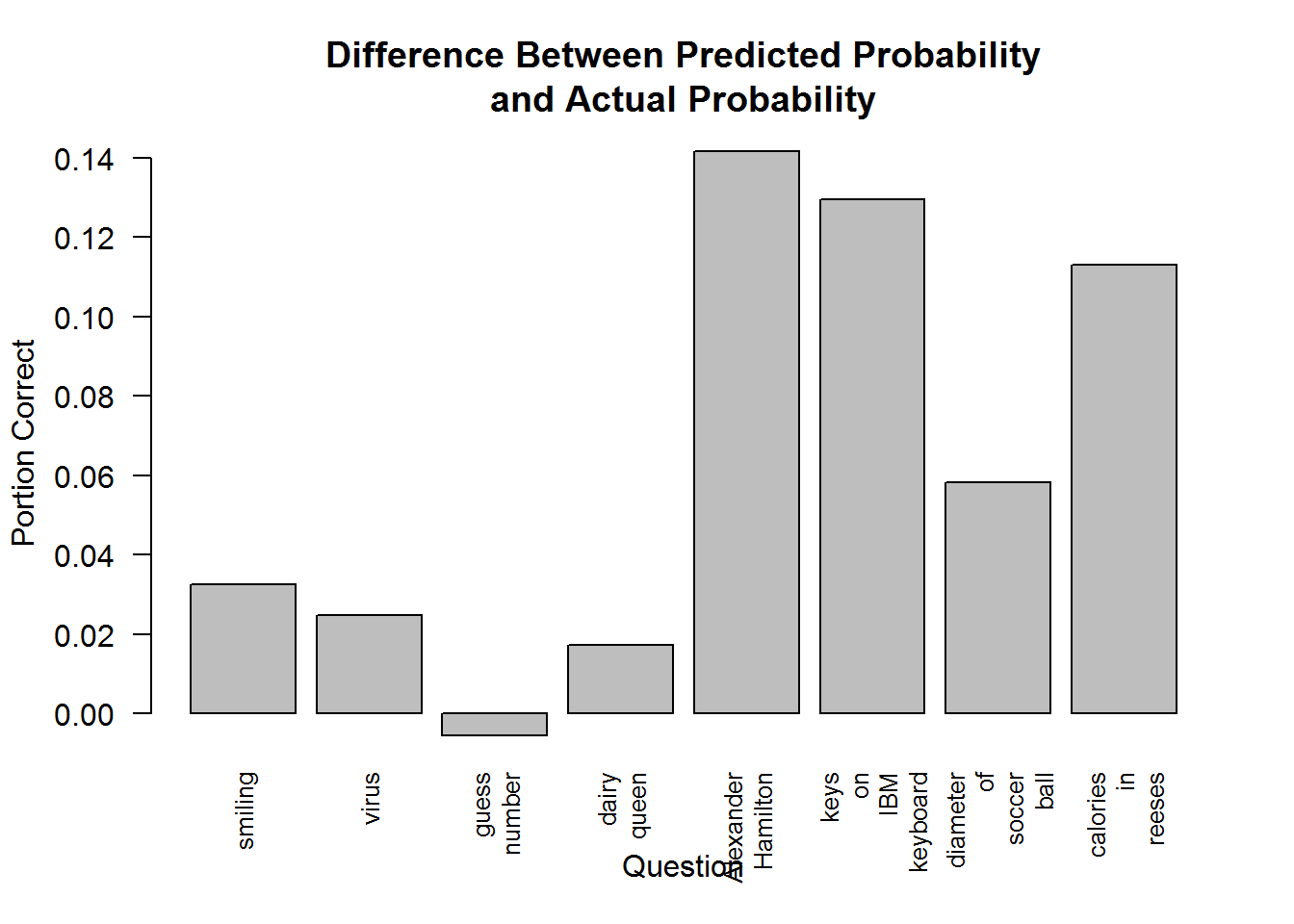Notice the scale of the graph is much smaller. People seem less overconfident when the probabilities are properly normalized, but we are overconfident on almost all of the questions.

The normalized probabilities are a bit harder to interpret. If someone predicted there was only a 10% chance they were right, it changes it to a prediction of a 90% chance they are wrong. Hence why the "guess a number" question is the only one where people are underconfident - they aren't predicting a high enough probability that they are wrong!

This has a weird effect on the smiling question. The median person of course had a probability of 1 for that. However some people (trolls?) put weird answers below 50% that got normalized, so about 3.2% of people were wrong about whether or not they were smiling. I should probably exclude those people from the analysis, but lets continue.

In one last attempt to define a measure of calibration, I have a weird idea. The normalized probability has some nice properties, but one problem is that it behaves weirdly around probability estimates of 0.5. It's impossible to be overconfident at 0.5. If you are overconfident that X is true, you can't also be overconfident that ~X is true. Probability of 0.5 represents maximum uncertainty and underconfidence. So probabilities above 0.5, should not pushed to probabilities less than 0.5, when adjusting for overconfidence.

An ad hoc way to fix this, is to come up with an arbitrary function that asymptotes at both 0.5 and 1. That's easy to do, the logistic function we used above for log odds actually has that exact property, if you transform the numbers from the range [0.5,1], to the range [0, 1], and then back when you are done. Lets write a function to do that:

``````#the logOdds function is just the logistic function, which transforms numbers from the range [0,1]
#to [-Infinity, Infinity], which is useful here. I adjust so it works on the range [0.5, 1] instead.
normprob2real <- function(p) logOdds((p-0.5)*2)
real2normProb <- function(r) logOdds2Prob(r)/2+0.5

function(ans, probs){
rprop(function(x){
geoMean(
getProbAssigned(
ans,
)
)
}, 1, 100)
}, normAns, normProbs)

``##  -0.1839873``
``````adjustProbsReal <- function(probs, optimalAdjust){
}

``##  0.6190165``

Wow, 0.62 GML is even worse than the improvement we got by normalizing the probabilities. I'm not confident this method is very good, even if it seems more sound in theory. This method suggests that if the median Lesswronger predicts something with 0.75 probability, they should decrease their estimate to 0.7. Or that we are overconfident by 5%. For comparison the first method suggests we are overconfident by 17% at the 0.75 mark, and the second suggests we are overconfident by just 3%.

## True Calibration

Ok so one of the issues mentioned above was that everyone seems to have about the same probability of getting any specific question right, and that most of the variation is just noise. If this is the case our calibration measures will also be very noisy as result.

We can fix this by taking that information into account. The below code redoes all the previous calibration measures, but with the assumption that there is a "true" probability for getting each question correct.

``````trueOptimalAdjust <- apply(completeProbs, 1,
function(probs){
rprop(function(x){
geoMean(
likelihood(
probsEachQuestion
)
)
}, 1, 100)
})

``##  -0.3051203``
``````trueOptimalAdjust2 <- apply(completeProbs, 1,
function(probs){
trueProbs <- ifelse(probs>0.5, probsEachQuestion, 1-probsEachQuestion)
probs <- ifelse(probs>0.5, probs, 1-probs)
rprop(function(x){
geoMean(
likelihood(
trueProbs
)
)
}, 1, 100)
})

``##  -0.1266545``
``````trueOptimalAdjust3 <- apply(completeProbs, 1,
function(probs){
trueProbs <- ifelse(probs>0.5, probsEachQuestion, 1-probsEachQuestion)
probs <- ifelse(probs>0.5, probs, 1-probs)
rprop(function(x){
geoMean(
likelihood(
trueProbs
)
)
}, 1, 100)
})

``##  -0.359464``
``````GMLMatrix <- completeProbs
GMLMatrix[ rep_len(TRUE, length(GMLMatrix)) ] <- mapply(likelihood, as.list(data.frame(completeProbs)), probsEachQuestion)
trueGMLs <- apply(GMLMatrix, 1, geoMean)``````

## Results

Such wildly different estimates from very similar methods is surprising, and it's not clear at all what method is best. One way to determine the best method, would be to look for correlations in the rest of the survey data. If one method of scoring calibration is highly correlated with, say, IQ, that would mean it's measuring some underlying calibration-ness ability better.

So I am going to write some code to test a large number of possible correlations between different features. I also want to test different types of normalization, because these numbers can have really weird distributions that might screw up the correlation tests. I expect that the best method of calibration will dominate, appearing most on the top of the list of correlations.

``````#normalize converts a vector in any distribution to a vector uniformily distributed between 0 and 1.
normalize <- function(vec) (rank(vec, na.last='keep')/sum(!is.na(vec))-(1/(sum(!is.na(vec))*2)))
#logitNormalize fits it to a logistic distribution. Sort of like how IQ is normalized to a gaussian distribution.
#This is useful because it places more empthasis on the differences between extreme cases.
#Moving between the 99th and 99.9th percentile becomes a much larger move than between 50 and 51 percentiles.
logitNormalize <- function(vec) logOdds(normalize(vec))

#making a list of all the normalize functions, including identity, because I want to test which one works the best
normFuncs <- list(function(n){n}, normalize, logitNormalize)
names(normFuncs) <- c("identity", "normalize", "logitNormalize")

#remove the rows of the survey that didn't fill out all the calibration questions
survey2 <- survey[calNotMissing, ]

#Geometric Mean of Likelihood, or how much probability they assigned the correct answers.
GMLs <- getGML(completeAns, completeProbs)

#how many answers they predicted they would get correct
meanProb <- apply(completeProbs, 1, mean)

#how different each person's meanProb assignment was, relative to the percent of questions the average person got right.
distanceFromAverage <- abs(meanProb-mean(completeAns))

#how different each person's meanProb is from the portion of questions they personally got correct
distanceFromTrue <- abs(meanProb-apply(completeAns, 1, mean))

testCols = c("IQ", "Age", "SAT", "SAT2", "ACT")

results <- matrix(nrow=length(testCalMeasures), ncol=length(testCols), dimnames=list(names(testCalMeasures), testCols))
for (col in testCols){
for (scol in names(testCalMeasures)){
score <- testCalMeasures[[scol]]
best <- 0
for (fn1 in 1:length(normFuncs)){
for (fn2 in 1:length(normFuncs)){
f1 <- normFuncs[[fn1]]
f2 <- normFuncs[[fn2]]

correlation <- cor(f1(survey2[[col]]), f2(score), use = "complete.obs")
if (abs(correlation) > abs(best))
best <- correlation
}
}
results[scol, col] <- best
}
}
results <- cbind(results, Mean = apply(abs(results), 1, mean))
results <- rbind(results, Mean = apply(abs(results), 2, mean))

library(ReporteRs)``````
``## Loading required package: ReporteRsjars``
``````#resFT <- FlexTable(round(results, 3), add.rownames = TRUE)
resFT <- vanilla.table(round(results, 3), add.rownames = TRUE)

resFT[,'Mean', side = 'left'] <- borderProperties( style = 'dashed' )
resFT['Mean', side = 'top'] <- borderProperties( style = 'dashed' )

scaleColor <- function(n, min, max){
gr <- (n-min)/(max-min)
rgb(1-gr, 1, 1-gr)
}

tableColors <- abs(results)
#tableColors[] <- normalize(tableColors)
tableColors[] <- scaleColor(tableColors, min(tableColors), max(tableColors))

resFT = setFlexTableBackgroundColors(resFT, i = 1:nrow(results), j = 2:(ncol(results)+1),
colors = t(tableColors))

#print(resFT)``````
``cat(as.html(resFT))``
 IQ Age SAT SAT2 ACT Mean optimalAdjust -0.040 0.110 -0.126 -0.161 -0.081 0.104 trueOptimalAdjust -0.075 -0.079 -0.135 -0.171 -0.134 0.119 optimalAdjust2 -0.052 0.062 -0.109 -0.194 -0.089 0.101 trueOptimalAdjust2 0.027 0.079 -0.106 -0.171 -0.134 0.103 optimalAdjust3 0.045 0.040 -0.079 -0.176 -0.097 0.088 trueOptimalAdjust3 -0.026 0.065 -0.099 -0.163 -0.131 0.097 GMLs 0.034 -0.069 0.165 0.128 0.077 0.094 trueGMLs -0.045 -0.111 0.114 0.177 0.145 0.118 meanProb 0.154 0.142 0.077 0.055 0.074 0.100 distanceFromAverage 0.122 0.133 0.084 0.032 0.033 0.081 distanceFromTrue 0.047 -0.034 -0.097 -0.017 -0.117 0.062 Mean 0.061 0.084 0.108 0.132 0.101 0.097

Alright let me explain the measures briefly:

• OptimalAdjust is the first measure I tried, that measures how much you should increase your probabilities/log-odds to get the optimal likelihood.
• OptimalAdjust2 is the second measure I tried, that is the same thing, but normalizes probabilities. E.g. a probability of 1% chance that you got the question right, becomes a 99% chance you got it wrong.
• OptimalAdjust3 is the ad hoc measure that I tried, which I thought would work the best. It normalizes the probabilities like above, and asymptotes the adjustment at 0.5. So that you can not be less confident than a 50% chance of true/false, or maximum uncertainty.
• GMLs is just Geometric Mean of Likelihood as explained above, that captures how good you were at predicting whether your answers would be correct or not.
• meanProb is a very simple measure, just how many questions you expected to get right, or your average confidence.
• distanceFromAverage is the absolute value of how different your meanProb is from the average percentage of questions answered correctly. The idea being that everyone had the same probability of getting any given question right, so people with higher or lower probabilities than the average were less calibrated.
• distanceFromTrue is the same principle, just the difference between the portion of questions you personally got correct.
• The trueX measures were all the measures that accounted for the fact that everyone had the same probability of answering each question correctly.

The best correlation seems to be between the second calibration measure I tried, and SAT2. But this seems to be a fluke. On average the first method seems to do the best. The trueX methods are always superior to the original measures. trueGML is the second best method and correlates better than optimalAdjust on several important things.

I also think there were not enough questions to get a completely accurate measure of calibration. Besides the smiling question, most people only got 1 question correct, and a significant percentage didn't get any. That might really affect any measure of calibration. And as we showed above, it seems like everyone was about equally likely to get any given question correct, so most of the variation in results comes down to random chance. But there are statistical correlations that are interesting.

One interesting thing is there is a distinction in the correlation chart between test scores and IQ and Age. Test scores correlate well with my various calibration scores, but IQ and Age correlate well with meanProb. The more questions you expected to get right, the higher your IQ and age. So higher IQ people and older people are more confident. Though that doesn't necessarily suggest they are overconfident. Lets see if they were:

``````#Correlation between Age and portion of questions correctly answered.
print(cor(normalize(survey2\$Age), apply(completeAns, 1, mean), use = "complete.obs"))``````
``##  0.1539956``
``````#Correlation between IQ and portion of questions correctly answered.
print(cor(normalize(survey2\$IQ), apply(completeAns, 1, mean), use = "complete.obs"))``````
``##  0.1275863``

So there is an equivalently strong correlation between IQ/Age and correctly answering the trivia questions. It's not that they are just overconfident.

OK one last way of visualizing this data is to plot the questions on a scatterplot, by the probability people expected to get them right, and how many actually got them right.

``````plot(apply(completeProbs, 2, median), probsEachQuestion, main="Scatterplot of Questions by\nEstimated Probability vs Actual",
xlab="Median Probability Assigned to Question", ylab="Portion of Respondents Correct", pch=19)
abline(0, 1)``````